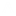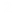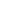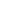```
1 {-
2
3 Pearl 2: Surpassing
4
5 Definitions:
6 A surpasser of an element of an array (list) is a greater element to the right.
7 x[j] is a surpasser of x[i] if i<j and x[i]<x[j].
8 The surpasser count of an element is the number of its surpassers.
9
10 Problem:
11 Compute the maximum surpasser count of an array of length n>1 in an O(n log n) algorithm.
12
13 Solution:
14
15 Note 1: In the imperative programming, using iterative+binary search.
16 We will use Divide and Conquer
17
18 Note 2: Cannot do better than O(n log n), b/c this is equivalen to sorting (you will see:
19 the table we create)
20 -}
21 module P2 where
22
23 -- Test word is:         GENERATION
24 -- Surpasser count is:   5625140100
25 -- So the maximum isL    6
26 testX = "GENERATION" :: [Char]
27
28 -- Maximum Surpasser Count
29 -- Brute force approach
30 -- Requires O(N^2) steps
31 -- Goes on shrinking list of length n: n+(n-1)+(n-2)+.. = n^2/2
32 msc         ::     Ord a => [a] -> Int
33 msc xs        =    maximum [scount z zs | z:zs <- tails xs]
34 scount x xs    =     length (filter (x<) xs)
35
36 -- We'll redfine tails, to make sure empty returns empty
37 tails             :: [a] -> [[a]]
38 tails []        = []
39 tails (x:xs)    = (x:xs):tails xs
40
41 -- Divide and Conquer
42 -- The trick is to find some 'data' that we can join together
43 -- easily. Then we can divide, and then join.
44 -- There are a few tricks to get to this 'join':
45 -- 1. Define data structure that contains enough information. Then you can join them.
46 --    This data structure is the table, that contains not only the Maximum Surpasser from
47 --    each divide, but all the counts!
48 -- 2. If we keep the Table in sorted order, the join can be done easily! (in Linear time)
49
50 table [x]        =    [(x,0)]        -- one element
51 table xs        =    join' (m-n) (table ys)  (table zs)
52                     where
53                         m = length xs
54                         n = m `div` 2
55                         (ys,zs) = splitAt n xs
56
57 join' 0 txs []    = txs
58 join' n []  tys    = tys
59 join' n txs@((x,c):txs') tys@((y,d):tys')
60         | x<y     = (x,c+n) : join' n txs' tys
61         | x>=y     = (y,d) : join' (n-1) txs tys'
62 -- the lists are ordered within themselves.
63 -- so if x<y ==> All the rest (and there's length n of tys) are also
64 -- greater than x ==> we should add this count!
65 -- if x>y, we just put y.
66 -- if x==y, we just want to make sure we continue in reduced manner, so we put (y,d), remmove
67 -- it from the next join, and then move on to continue.
68
69 -- Main
70
71 main = do
72     putStr "Brute force       : "
73     print \$ msc testX
74     putStr "Divide and Conquer: "
75     print \$ maximum \$ map (snd) (table testX)
```